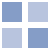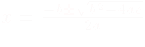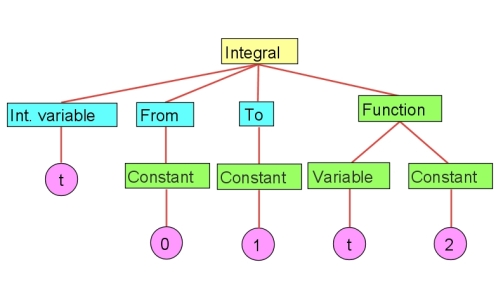12 Aug 2005

# MathML

These slides are in XHTML, and follow the approach described on a separate page for presentation. That page describes the minimum environment you should have to display these slides with the proper MathML content.

# Why MathML?

Current solutions to display math on the Web:

Use HTML
eg: ai (`a<sub>i</sub>`))
• HTML is not rich enough
• typesetting mathematical formulae is hard!
Use embedded images
eg:• requires specialized editors
• difficult to position/size the image properly
• not searchable
• accessibility problems (what should a voice browser do?)

# Why MathML? (cont.)

• Mathematics are ubiquitous in technical documentation
• Having mathematics on the web is essential for digital documents!
• Large amount of legacy document should be converted (mostly in TEX)
• Hence MathML…

# What is MathML?

• Mathematical formulae described in XML
• it is searchable
• can be embedded in other, XML-based markups
• is human editable (sort of… specialized editors are better)
• Reminiscent to TEX (ie, converter tools are feasible)

# Who developed MathML?

• Elsevier, INRIA, Univ. of Waterloo (Maple), IBM, American Mathematical Society, the OpenMath consortium, Wolfram Research (Mathematica),…
• Software are now available:
• IBM Techexplorer, or MathPlayer plugins
• Firefox displays MathML natively
• DesignSite with MSN
• Mathematica and Maple export and render MathML
• LaTeX2HTML MathML and Omega packages convert TEX to MathML

# A Flavour of MathML: Quadratic formula in MathML

 `` ` x =` ` ` ` ` ` -b` ` ±` ` ` ` ` ` b2` ` -` ` ` ` c` ` ` ` ` ` ` ` ` ` ` ` a` ` ` ` ` ``

# A Bit of History

• MathML 1.0 was published in 1998 with an update in 1999
• MathML 1.0 was the first XML based W3C Recommendation!
• MathML 2.0 was published in 2002, with an update in 2003
• “modernized” MathML 1.0 for the evolution of XML
• styling, namespaces, usage of Unicode, etc.
• MathML 2.0, 2nd. edition is the authoritative version!

# MathML structure

XML Elements in MathML are:

Presentation markup elements:
Describing the notation directly (see previous example): rows, fractions, operators, etc. It captures the notational structure.
Content markup elements:
Explicit encoding of the mathematical content. It captures the mathematical structure.
The (only) interface element:
`math` element, which binds a formula to the surrounding XML (eg., XHTML)

Note: earlier versions relied on XML Entities for special signs (integrals, greek letters, etc), but Unicode has taken over…

# Same Formula in Different Markups

Presentation Markup Content Markup
`<msup>`
`  <mfenced>`
`    <mrow>`
`      <mi>a</mi>`
`      <mo>+</mo>`
`      <mi>b</mi>`
`    </mrow>`
`  </mfenced>`
`  <mn>2</mn>`
`</msup>    `
`<apply>`
`  <power/>`
`  <apply>`
`    <plus/>`
`    <ci>a</ci>`
`    <ci>b</ci>`
`  </apply>`
`  <cn>2</cn>`
`</apply>`

# Why two markups?

• Legacy data transformed into presentation markup
• Authoring tools may prefer presentation markup
• High quality renderers may prefer to use content markup:
• difference between an inline vs standalone formula
• differences in local traditions, disciplines:
• 3+4i resp. 3+4j!
• Pedagogic tools would use content markup
• Content markup represents abstract information
• Content markup is more accessible
• The two markups may be mixed!

# Presentation Elements

Presentation elements fall in two categories:

Token elements
Individual symbols, names, numbers, alignment control, ...
Layout elements
Rows, fractions, square roots, superscripts, tables, ...

# Main Token Presentation Elements

 identifier, number `a 2` operator, separator, or accent `ab` text `Theorem 1:`

# Controlling the Rendering

Attributes to control the precise rendering, eg,

 ` ( ` ` ( `

# Controlling the Rendering (cont.)

• Other examples:
• font size, color, …
• leftspace, rightspace, …
• operator form (infix, prefix, postfix)
• operator dictionary for the characterization of the operators
• invisible operators (eg, "invisible times"), important for, eg, voice browsers!

# General Layout

 subexpressions `ab` fraction `ab` square root `a`

# General Layout (cont.)

 radical `ab` surround content `` ` ab` ``

# Script and Limits

 subscript `xi` superscript `xi` tensor indices `R` ` ijkl` ` ab` ` ...` ``

# Tables and Matrices

 table ` [ ` `` ` 1` ` 0` ` 0` `` `...` ` ] ` row entry

# Tables and Matrices (cont.)

 align `` ` ` ` ` ` 8.44 ` ` ` ` x ` ` ... ` ` ` ` ` ` ` ` 3.1 ` ` ` ` x ` ` ...` ` ` ``

# Enlivening Expressions

 `` ` ...` ` ...` ``

Other possible action types (depending on the rendering engine)

• tooltip
• statusline
• highlight background, foreground

# Content Markup

Content markup reflects the structure of formulae:# Content Markup Details

Content markup reflects the structure of formulae:

 ` ` ` t` ` 0` ` 1` ` ` ` t` ` 2` ` ` ` `

MathML does not define the exact mapping from content markup to rendering (only a "Default Rendering" is defined).

# Content Markup

• MathML defines a small number of commonplace mathematical constructs, covering
• arithmetic, algebra, linear algebra, logic and relations
• calculus, sequences and series, set theory
• trigonometry
• statistics
• Adequate for an A-level (US) or Baccalaureate (Europe) mathematics

# Fundamental Content Elements

 Number `` ` 34` `` Variable `` ` F` ` x` `` Apply construct Numeric interval `` ` 34` ``

# Fundamental Content Elements (cont.)

 Functions and operators `` ` f` `` Inverse function `` ` ` ` x` ``

# Fundamental Content Elements

 Condition `` ` ` ` ` ` ` ` ` ` x` ` B` ` ` ` ` ` x` ` C` ` ` ` ` ` ` ` x` ``

# Fundamental Content Elements

 Matrices `` ` ` ` 1 2 ` ` ` ` ` ` 3 4 ` ` ` `` Piecewise `` ` ` ` ` ` x ` ` ` ` ` ` x ` ` 0 ` ` ` ` ` ` ...` ``

# Content Operators and Functions

Arithmetic, Algebra, Logic
`quotient, exp, max, min, root, and, xor, forall, exists, floor` ...
Relations
`eq, neq, equivalent,` ...
Calculus
`ln, int, partialdiff, diff, limit, laplacian` ...
Set theory
`list, union, intersect, cartesian product` ...

# Content Operators and Functions

Sequences and series
`sum, product,` ...
Statistics
`mean, variance, moment` ...
Trigonometry
`sin, arccos,` ...
Linear algebra
`vector, matrix, vector product,` ...
Constants
`integers, reals, pi, infinity,` ...

Altogether cca. 100 elements!

# Mixing Content and Presentation Markups

• Content markup helps accessibility (eg, voice reader); but …
• …it cannot account for all situations! For example:
 ` ` ` ` ` ` ` F` ` i` ` ` ` ` ` x` ` `

# Semantics of Content Elements

• Elements can use the `definitonURL` to override default semantics (eg, infix, postfix)
• Recommendation includes a formalism for defining semantics
• Other specifications through `csymbol`:
`<!-- reference to OpenMath definition of Bessel function -->`
`<apply>`
`  <csymbol encoding="OpenMath"`
`    definitionURL="http://www.openmath.org/...">`
`    <msub><mi>J</mi><mn>0</mn></msub>`
`  </csymbol>`
`  <ci>y</ci>`
`</apply>`

# Adding semantics to an expression

`<semantics>`
`  <apply>`
`  <divide/>`
`    <cn>123</cn>`
`    <cn>456</cn>`
`  </apply>`
`  <annotation encoding="Mathematica">`
`       N[123/456, 39]`
`  </annotation>`
`  <annotation encoding="Maple">`
`       evalf(123/456, 39);`
`  </annotation>`
`  <annotation-xml encoding="OpenMath">`
`    <OMA xmlns="http://www.openmath.org/OpenMath">`
`	  <OMS cd="arith1" name="divide"/>`
`	  <OMI>123</OMI>`
`	  <OMI>456</OMI>`
`    </OMA>`
`  </annotation-xml>`
`</semantics>`

# Using MathML with Other Markups

• The `math` element:
• “root” element of MathML
• has attributes to control font, colour, background colour, overflow control,etc,
• XSLT can also be used to “transform” markups on the fly:
• adapt to local conventions (“tan” vs “tg”, “[a,b]” vs “]a;b[”, etc)
• is a powerful technology that MathML “inherits” from other XML recommendations

# Embedding MathML

• Usage of `embed`:
`<embed src="mmls/mixExample.mml" height="60" width="110" />`
• Non-standard tag
• Not all browsers may support it
• Might be the only way to use with some plugins… (sigh!)

# Using “object”

• Alternative should be `object`:
`<object type="application/mathml+xml"`
`   data="mmls/mixExample.mml"`
`   height="60" width="110"></object>`
• Proper HTML4 and XHTML tag
• The `iframe` can also be an alternative in some cases…

# Full XML Based Usage: Namespaces

Using XHTML, the math content should be directly includable:

`<body>`
`  <p>Bla bla bla</p>`
`  <m:math xmlns:m="http://www.w3.org/1998/Math/MathML">`
`    <m:mrow>...<m:mrow>`
`  </m:math>`
`</body>`
• Works in Firefox (and derivatives), in Amaya, in Gaelon
• content markup may not be implemented
• content must be served as xml, not as text/html
• IE 6.+ with MathPlayer or TechExplorer plugins
• file has to follow IE’s behavior rules…
• There is a separate Working group at W3C cleaning up this approach (Compound Document Formats)

# Full XML Based Usage: Common Approach

• MathML activity has developed some XSLT scripts to unify IE and Mozilla/Netscape
• “Universal Math XSL stylesheet (UMSS)”
• Same file can be displayed on both platforms
• the IE “behavior” requirements are hidden, for example
• if necessary content markup is transformed into presentation markup “on the fly”
• Caveat (again): the file must be served as XML and not in HTML
• This is how the file should look like:
`    <?xml version="1.0" encoding="iso-8859-1" ?>`
`    <?xml-stylesheet type="text/xsl" href="mathml.xsl"?>`
`    <html xmlns="http://www.w3.org/1999/xhtml">`
`      <head>`
`        …`

# Further Information

These slides are at:
http://www.w3.org/Consortium/Offices/Presentations/MathML/
W3C MathML Activity Page:
http://www.w3.org/Math/
The latest release of the Recommendation
http://www.w3.org/TR/MathML2/